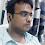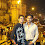# NVIDIA Interview Questions

You are designing a circuit that implements two operations A and B as shown below.NOTES:

1. At any point in time the circuit is doing either A or B.
2. Delays through modules of each operation are given in the figure below.
3. The circuit must have registers on all inputs. No registers are needed on the outputs.
4. The delay through a register is 5ns.
5. Operation A occurs 70% of the time while operation B occurs 30% of the time.

What is the clock period that will result in highest overall performance?

And questions increasing in difficulty.

1.Assuming the internal modules cannot be further pipelined, the clock would have to be greater than 50 + 5 = 55 ns. This is assuming registers are put in between all the internal modules.
I don't see the relevance of the 70-30 division, as we have to take the critical path into account. What am I missing?

2.Higher the frequency, higher will be the performance. But we need to check what is the minimum delay being taken by any unit in the design. All "input registers" are taking a delay for "5 ns". So the system clock should be designed taking this into consideration. Other informations are irrelevant.

3.As there is no delay needed at output so 5ns register delay will not come in picture. Because within the 20ns+5ns period the processing unit "G" can put the correct data into the unit "h" so the clock period will be 50ns and not 50ns + 5ns.
Also, looking from other angle. if 50ns is the period all the internal pipeline units (f,g,k) can put the data properly in next unit (g,h,l respectively) for the pipeline to function perfectly. If there is a output delay requirement from our critical path unit (i.e. h) then that delay will add up into the clock period.

4.I think it should be 50ns, not 55.

5.for detail solution mail me
mr.saurabh.srivastava@gmail.com

6.It should be 55ns ( 50+5)

7.Ok Ok
Guys lets cross check
By 55 clock period (denoted by C5)
scheduling would be
Stages - 45(40+5) 25(20+5) 55(50+5)
C5 will take 3 cycle ok
Stage 35 , 25 (for operation B)
C5 will take 2 clock cycle
Total average time
55*(0.7*3+0.3*2)= 148.5

Now C3 clock with 35 period
Operation A 5 clock
Operation B 2 clock
Total average time
35*(0.7*5+0.3*2)= 143.5

Clear now

8.Yes, but with 35ns clock, the register at g input will never receive the output from f before the next clock tick. The clock period has to be sufficient to accommodate for the maximum combinational path delay in the pipe stages and the setup/hold time of the register. The delays in each module are a clear indication that they are combinational and cannot be split further. I agree with Mk's answer. the register setup time will be added to the previous stage's delay. Also, nothing has been said about the output of h. So if we assume the output to be left as a combinational one (not connected to any FF), then the clock period will be 45ns. Although this will cause a warning during par.

9.10.11.As #8 said, the clock period can't be < 45 or else you'll lose the value at g. The latency is shortest per operation if tclk >= 115 ns, but the throughput suffers. The throughput is best if you use the shortest allowable clock, which in this case is 45 since you don't have to register the outputs. If you want to optimize for the best latency/throughput compromise, you'd need to know what the questioner means by "overall performance".

12.45 ns, due to f()+reg
or:
5 ns, the gcd of all times present, where we have to use additional logic to decide when to sample and when not.

If we are allowed 35 ns, for k()+reg, using 2 cycles at f() and h(), the percentages will be interesting, else not, but then again, 5 ns is even better, like perfect ;-)

13.SaurabhS, I agree with your argument, but the correct answer is 30ns. If you go by the same logic, 30ns gives you 30*(6*0.7+4*0.3)=132ns per instruction on an average.

14.The answer will be 55ns. The minimum clock period for correct functioning of the circuit is given by:
tclk >= t(clk-Q) + tlogic(max) + tsetup
here t(clk-Q) is the delay of the output to appear at the output (Q) of the pipeline register, tlogic(max) is the maximum delay in the circuit implementing the logic (remember it is not the minimum time because we have to consider the worst case logic delay in order to guarantee correct functionality) and tsetup is the time before the next clock edge that the data to be latched has to be stable. Here as there is no output register in the last module of operation A, the optimum tclk = 5ns(t(clk-Q)) + 50ns(tlogic(max)) = 55ns